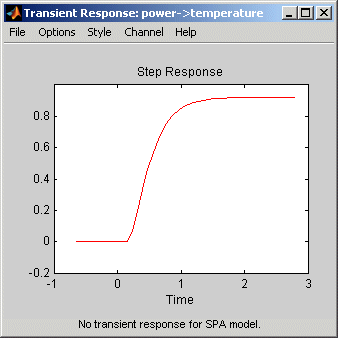Main Content

## Impulse and Step Response Plots

### Supported Models

You can plot the simulated response of a model using impulse and step signals as the input for all linear parametric models and correlation analysis (nonparametric) models.

You can also create step-response plots for nonlinear models. These step and impulse response plots, also called transient response plots, provide insight into the characteristics of model dynamics, including peak response and settling time.

Note

For frequency-response models, impulse- and step-response plots are not available. For nonlinear models, only step-response plots are available.

### How Transient Response Helps to Validate Models

Transient response plots provide insight into the basic dynamic properties of the model, such as response times, static gains, and delays.

Transient response plots also help you validate how well a linear parametric model, such as a linear ARX model or a state-space model, captures the dynamics. For example, you can estimate an impulse or step response from the data using correlation analysis (nonparametric model), and then plot the correlation analysis result on top of the transient responses of the parametric models.

Because nonparametric and parametric models are derived using different algorithms, agreement between these models increases confidence in the parametric model results.

### What Does a Transient Response Plot Show?

Transient response plots show the value of the impulse or step response on the vertical axis. The horizontal axis is in units of time you specified for the data used to estimate the model.

The impulse response of a dynamic model is the output signal that results when the input is an impulse. That is, u(t) is zero for all values of t except at t=0, where u(0)=1. In the following difference equation, you can compute the impulse response by setting y(-T)=y(-2T)=0, u(0)=1, and u(t>0)=0.

The step response is the output signal that results from a step input, where u(t<0)=0 and u(t>0)=1.

If your model includes a noise model, you can display the transient response of the noise model associated with each output channel. For more information about how to display the transient response of the noise model, see Plot Impulse and Step Response Using the System Identification App.

The following figure shows a sample Transient Response plot, created in the System Identification app.### Displaying the Confidence Interval

In addition to the transient-response curve, you can display a confidence interval on the plot. To learn how to show or hide confidence interval, see the description of the plot settings in Plot Impulse and Step Response Using the System Identification App.

The confidence interval corresponds to the range of response values with a specific probability of being the actual response of the system. The toolbox uses the estimated uncertainty in the model parameters to calculate confidence intervals and assumes the estimates have a Gaussian distribution.

For example, for a 95% confidence interval, the region around the nominal curve represents the range where there is a 95% chance that it contains the true system response. You can specify the confidence interval as a probability (between 0 and 1) or as the number of standard deviations of a Gaussian distribution. For example, a probability of 0.99 (99%) corresponds to 2.58 standard deviations.

Note

The calculation of the confidence interval assumes that the model sufficiently describes the system dynamics and the model residuals pass independence tests.

## SupportGet trial now